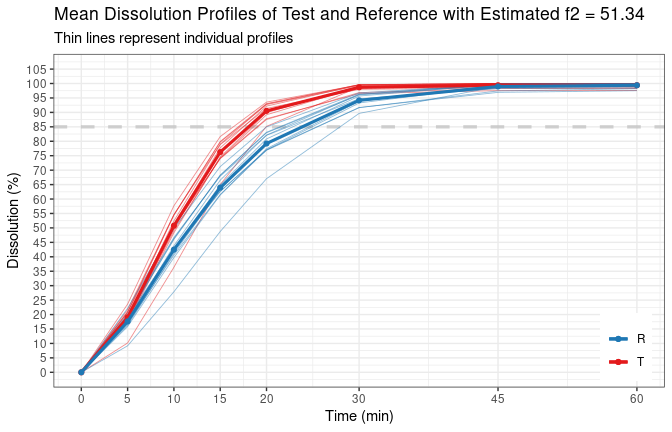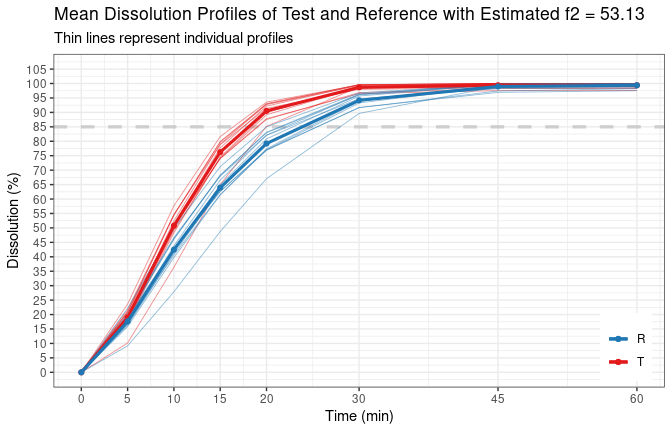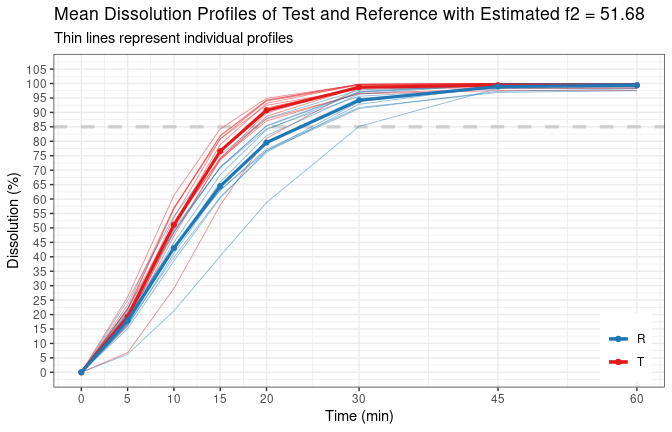# Calculating Similarity Factor $$f_2$$

## Introduction

To use traditional $$f_2$$ method, several conditions defined in the regulatory guidelines have to be fulfilled. Different guidelines phrase the conditions differently, which led to confusion some times. The details were explained in the vignette Introduction to bootf2.

This document gives some examples of the usage of the function calcf2().

## Usage

The complete list of arguments of the function is as follows:

calcf2(test, ref, path.in, file.in, path.out, file.out,
regulation = c("EMA", "FDA", "WHO", "Canada", "ANVISA"),
cv.rule = TRUE, message = FALSE, min.points = 3L,
f2.type = c("est.f2", "exp.f2", "bc.f2", "vc.exp.f2",
"vc.bc.f2", "all"), both.TR.85 = FALSE,
digits = 2L, time.unit = c("min", "h"),  plot = TRUE,
plot.start.time = 0, plot.max.unit = 24L)

### Notes on function arguments

1. Data input: test, ref, path.in, file.in
• In the typical interactive use, test and ref are data frames with the time as the first column, and individual units for the rest of columns. In such cases, arguments path.in and file.in should not be used.
• Data can be read directly from an Excel file with extension .xlsx or .xls. In this case, data of test and reference should be stored in separate worksheets. The first column should be time, the rest columns are individual units. The first row is the column head indicating the names, such as ‘time,’ ‘unit 01,’ unit 02’, …. It doesn’t matter what the names are as columns will be renamed internally by the function. The important point is that the first row will not be read, so do not put dissolution data on the first row.
• When path.in and file.in are provided, the argument test and ref should be the worksheet names inside quotation mark, e.g., "lot ABCD1234 pH 6.8".
• path.in can be an absolute path such as"/home/myname/my.project/dat/", or a relative path such as "../dat/" if the working directory is in the folder "/home/myname/my.project/analysis/" and the data file is in the folder "/home/myname/my.project/dat/".
• One more note for Windows user: As Windows use “" instead of”/" to separate path, you have to either escape it by an additional "", e.g., "C:\user\myname\my.project\dat\" cannot be the path.in, you have to changed it to "C:\\user\\myname\\my.project\\dat\\", or to "C:/user/myname/my.project/dat/", the same format as used in Linux system.
2. For such simple calculation, argument path.out and file.out are really overkill. By default the result will be printed to screen. But if somehow you feel that you need it, the same principle for path.in and file.in applies here.
3. The $$f_2$$ value should be reported without decimal.
4. When argument cv.rule is set to TRUE, and if argument message is also TRUE, additional message will be printed to screen. One particular note is the rounding of CV before checking compliance. Since the guidelines does not specify the precision for CV, when checking if CV condition defined in the guidelines is fulfilled, the calculated CV values are rounded with digits decimal first before they were compared to the 20%/10% criterion. And refer to vignette Introduction to bootf2 for details of regulation rules.
5. In terms of how many time points to be used in the calculation (especially those points with dissolution more than 85%), the "EMA" and "FDA" are the same. See vignette Introduction to bootf2 for details. The reason both of them are included in regulation argument is because they have different rules for CV.
6. If the argument both.TR.85 = TRUE, and regulation = FDA, then the function will calculate $$f_2$$ using all time points until both test and reference dissolve more than 85%. This is the wrong interpretation of the guidelines, as explained in the vignette Introduction to bootf2. The purpose of this argument is for historical reason, in case users want to check the calculation published in the old articles where the wrong interpretation was used, or for validation purpose. It should be set to FALSE for the daily analysis.
7. For traditional $$f_2$$ calculation, do not specify the argument f2.type. This argument is only for bootstrap method. The default est.f2 is the correct one to use. See vignette Confidence Intervals of $$f_2$$ Using Bootstrap Method for details.
8. The argument plot.max.unit control how individual profile will be represented in the plot. When the actual number of units is greater than the value of plot.max.unit, the individual profile will be represented as boxplots at each time points.
9. For delayed-release formulation, it is typical that the product will be put in acidic media for 2 hours where almost no dissolution will occur, then transferred to different medium (e.g., pH 6.8 medium). In such special case, setting plot.start.time = 120 (or plot.start.time = 2 if the time unit is "h") for example, can make plots more readable.
10. Read the function manual by help("calcf2") for more details on each argument.

## Simple case with low variability

First, let’s simulate profiles with low variability for reference and test.

# time points
tp <- c(5, 10, 15, 20, 30, 45, 60)

# model.par for reference with low variability
par.r1.lv <- list(fmax = 100, fmax.cv = 3, mdt = 15, mdt.cv = 13,
tlag = 0, tlag.cv = 0, beta = 1.5, beta.cv = 7)

# simulate reference data
dr1.lv <- sim.dp(tp, model.par = par.r1.lv, seed = 100, plot = FALSE,
message = TRUE)
# Dissolution data was generated using Weibull model, with the
# following model parameters:
# - fmax      = 100
# - fmax.cv   = 3%
# - tlag      = 0
# - tlag.cv   = 0%
# - mdt       = 15
# - mdt.cv    = 13%
# - beta      = 1.5
# - beta.cv   = 7%
#
# Seed number used:  100

# model.par for test
par.t1.lv <- list(fmax = 100, fmax.cv = 3, mdt = 12.29, mdt.cv = 10,
tlag = 0, tlag.cv = 0, beta = 1.727, beta.cv = 8)

# simulate test data with low variability
dt1.lv <- sim.dp(tp, model.par = par.t1.lv, seed = 100, plot = FALSE,
message = TRUE)
# Dissolution data was generated using Weibull model, with the
# following model parameters:
# - fmax      = 100
# - fmax.cv   = 3%
# - tlag      = 0
# - tlag.cv   = 0%
# - mdt       = 12.29
# - mdt.cv    = 10%
# - beta      = 1.727
# - beta.cv   = 8%
#
# Seed number used:  100

Calculate f2 with default setting (following EMA’s guideline)

t_vs_r_ema <- calcf2(dt1.lv$sim.disso, dr1.lv$sim.disso,
message = TRUE)
# The f2 method was applied according to EMA's BE guideline.
#
# Individual data was provided with option 'cv.rule = TRUE',
# therefore, CV has been calculated and checked accordingly.
#
# Calculated mean and CV as follows:
# Time   Mean (T)   Mean (R)     CV (T)     CV (R)
# 5         19.02      17.60      17.66      18.33
# 10        50.77      42.48      10.46      12.82
# 15        76.24      63.95       5.79       8.95
# 20        90.52      79.22       2.91       5.99
# ------------------------------------------------
# 30        98.68      94.18       1.08       2.38
# 45        99.41      98.98       0.88       0.98
# 60        99.42      99.39       0.88       0.89
# ==================================
# Number of units for test is      : nt = 12
# Number of units for reference is : nr = 12
#
# CV criteria fulfilled; therefore, f2 method can be applied.
#
# The time points above the dashed line are used in f2 calculation.
#
# Estimated f2 = 51.34calculate f2 following WHO guideline

t_vs_r_who <- calcf2(dt1.lv$sim.disso, dr1.lv$sim.disso,
regulation = "WHO", message = TRUE)
# The f2 method was applied according to WHO's BE guideline.
#
# Individual data was provided with option 'cv.rule = TRUE',
# therefore, CV has been calculated and checked accordingly.
#
# Calculated mean and CV as follows:
# Time   Mean (T)   Mean (R)     CV (T)     CV (R)
# 5         19.02      17.60      17.66      18.33
# 10        50.77      42.48      10.46      12.82
# 15        76.24      63.95       5.79       8.95
# 20        90.52      79.22       2.91       5.99
# 30        98.68      94.18       1.08       2.38
# ------------------------------------------------
# 45        99.41      98.98       0.88       0.98
# 60        99.42      99.39       0.88       0.89
# ==================================
# Number of units for test is      : nt = 12
# Number of units for reference is : nr = 12
#
# CV criteria fulfilled; therefore, f2 method can be applied.
#
# The time points above the dashed line are used in f2 calculation.
#
# Estimated f2 = 53.13Calculate f2 following FDA guidance, to confirm “old calculation” with wrong interpretation. See vignette Introduction to bootf2 for details.

t_vs_r <- calcf2(dt1.lv$sim.disso, dr1.lv$sim.disso, regulation = "FDA",
both.TR.85 = TRUE, message = TRUE, plot = FALSE)
# The f2 method was applied according to FDA's BE guidance.
#
# Individual data was provided with option 'cv.rule = TRUE',
# therefore, CV has been calculated and checked accordingly.
#
# Calculated mean and CV as follows:
# Time   Mean (T)   Mean (R)     CV (T)     CV (R)
# 5         19.02      17.60      17.66      18.33
# 10        50.77      42.48      10.46      12.82
# 15        76.24      63.95       5.79       8.95
# 20        90.52      79.22       2.91       5.99
# 30        98.68      94.18       1.08       2.38
# ------------------------------------------------
# 45        99.41      98.98       0.88       0.98
# 60        99.42      99.39       0.88       0.89
# ==================================
# Number of units for test is      : nt = 12
# Number of units for reference is : nr = 12
#
# CV criteria fulfilled; therefore, f2 method can be applied.
#
# The time points above the dashed line are used in f2 calculation.
#
# Estimated f2 = 53.13*
#
# *Note: Argument 'both.TR.85' is 'TRUE', which is the wrong interpretation
# of the guidance. This should only be used for cases such as checking the
# calculation published in the old literature.

# Cases with high variability

Simulate profiles with the same population parameters but with higher variability.

# model.par for reference with high variability
par.r1.hv <- list(fmax = 100, fmax.cv = 3, mdt = 15, mdt.cv = 20,
tlag = 0, tlag.cv = 0, beta = 1.5, beta.cv = 10)

# simulate reference data
dr1.hv <- sim.dp(tp, model.par = par.r1.hv, seed = 100, plot = FALSE)

# model.par for test
par.t1.hv <- list(fmax = 100, fmax.cv = 3, mdt = 12.29, mdt.cv = 15,
tlag = 0, tlag.cv = 0, beta = 1.727, beta.cv = 12)

# simulate test data with low variability
dt1.hv <- sim.dp(tp, model.par = par.t1.hv, seed = 100, plot = FALSE)

When CV conditions are not fulfilled, the function will stop running.

calcf2(dt1.hv$sim.disso, dr1.hv$sim.disso, message = TRUE)
# The f2 method was applied according to EMA's BE guideline.
#
# Individual data was provided with option 'cv.rule = TRUE',
# therefore, CV has been calculated and checked accordingly.
#
# Calculated mean and CV as follows:
# Time   Mean (T)   Mean (R)     CV (T)     CV (R)
# 5         19.19      17.92      25.22      25.93
# 10        51.11      43.02      15.82      19.05
# 15        76.58      64.46       9.13      13.90
# 20        90.73      79.55       4.49       9.66
# ------------------------------------------------
# 30        98.70      94.20       1.18       3.83
# 45        99.41      98.95       0.88       1.05
# 60        99.42      99.39       0.88       0.89
# ==================================
# Number of units for test is      : nt = 12
# Number of units for reference is : nr = 12
#
# CV criteria not fulfilled; therefore, f2 method cannot be applied.
# Error in calcf2(dt1.hv$sim.disso, dr1.hv$sim.disso, message = TRUE): You should consider alternative methods such as bootstrap f2.

There might be a time that you just want to check the $$f_2$$ regardless of the variability, option cv.rule can be set to FALSE in such case.

dt1.hv_vs_dr1.hv <- calcf2(dt1.hv$sim.disso, dr1.hv$sim.disso,
cv.rule = FALSE, message = TRUE)
# The f2 method was applied according to EMA's BE guideline.
#
# Individual data was provided with option 'cv.rule = FALSE',
# therefore, CV has been calculated but has not been checked.
# You should really consider setting 'cv.rule = TRUE' to comply
# regulatory requirements.
#
# Calculated mean and CV as follows:
# Time   Mean (T)   Mean (R)     CV (T)     CV (R)
# 5         19.19      17.92      25.22      25.93
# 10        51.11      43.02      15.82      19.05
# 15        76.58      64.46       9.13      13.90
# 20        90.73      79.55       4.49       9.66
# ------------------------------------------------
# 30        98.70      94.20       1.18       3.83
# 45        99.41      98.95       0.88       1.05
# 60        99.42      99.39       0.88       0.89
# ==================================
# Number of units for test is      : nt = 12
# Number of units for reference is : nr = 12
#
# CV has not been checked.
#
# The time points above the dashed line are used in f2 calculation.
#
# Estimated f2 = 51.68